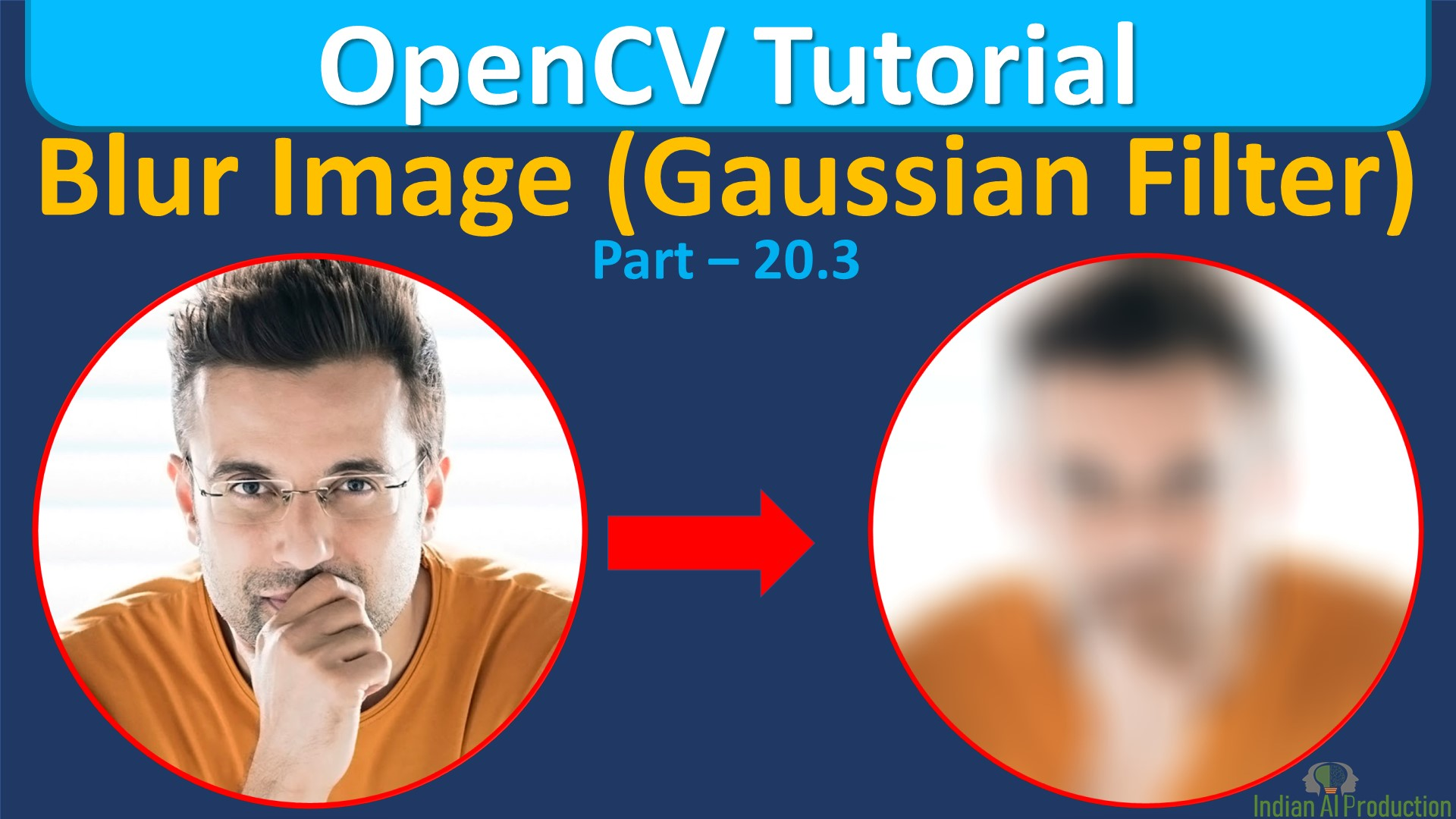## Blur Image using Gaussian Filter OpenCV Python | OpenCV Tutorial

In Python OpenCV Tutorial, Explained How to Blur image using cv2.GaussianBlur() opencv function. Get the answers of below questions: How do I blur an image in OpenCV?How do you blur an image in Python?Why do we blur image?How do you blur part of a picture? Syntax: cv2.GaussianBlur(src, ksize, sigmaX ]]) -> dst . @brief Blurs an image using a Gaussian filter. . . The function convolves the...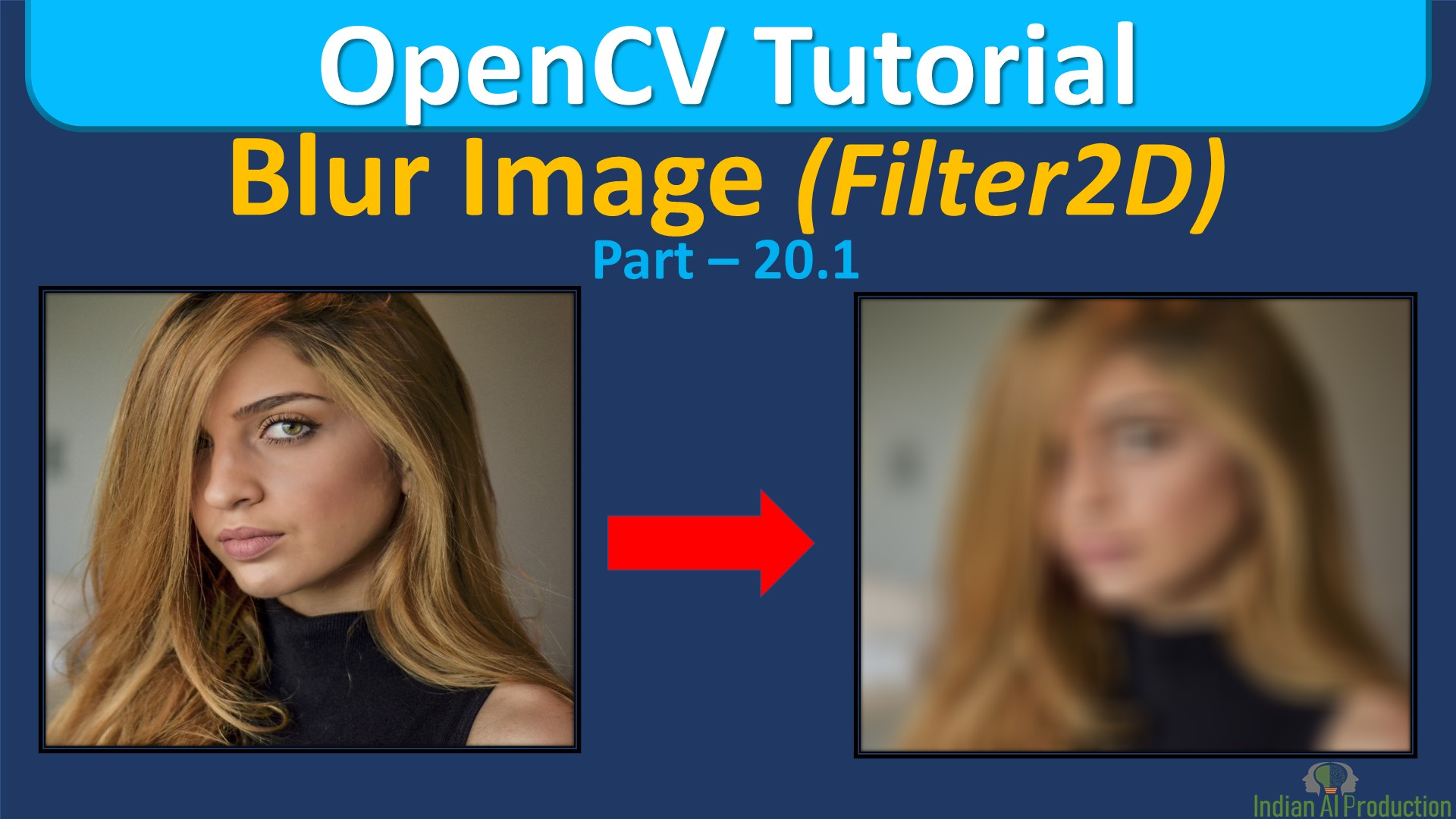## Blur Image using filter2d OpenCV Python | OpenCV Tutorial

In Python OpenCV Tutorial, Explained How to Blur image using cv2.filter2d() opencv function. Get the answers of below questions: How do I blur an image in OpenCV?How do you blur an image in Python?Why do we blur image?How do you blur part of a picture? Syntax: cv2.filter2D(src, ddepth, kernel ]]]) -> dst Parameters: . @param src input image. . @param dst output image of the same size and the...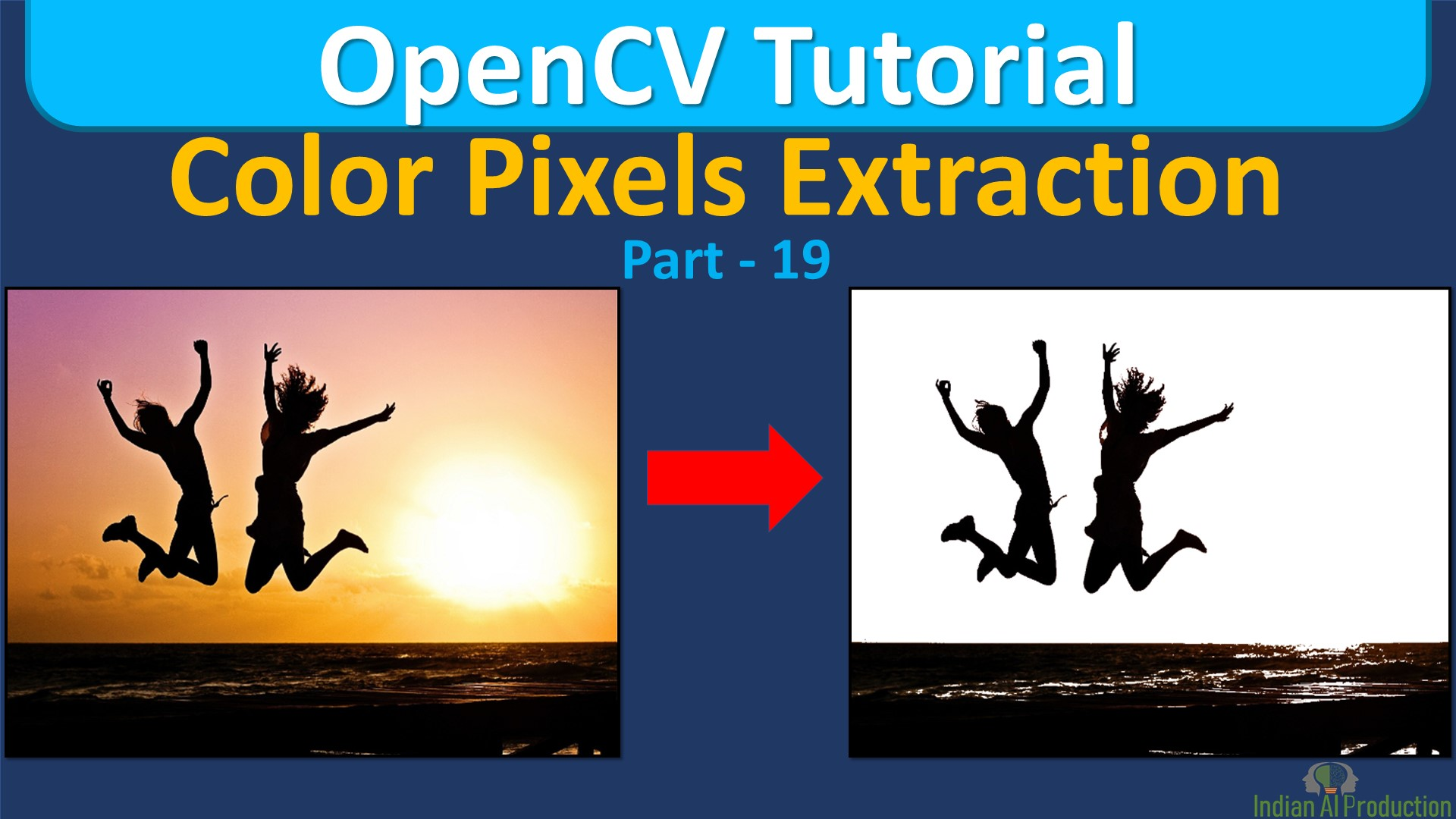## Color Pixels Extraction using OpenCV Python | OpenCV Tutorial

In Python OpenCV Tutorial, Explained How to Extraction or Detect Pixels Color using OpenCV Python Get the answers of below questions: How do you find the color of the pixel of an image?How do you find the color of an image in Python?How do I extract color features from an image?How do we find items of a specific color?How can I identify a color in an image? Color Pixels Extraction How to...## How to Show Histogram of Image using OpenCV Python | OpenCV Tutorial

In Python OpenCV Tutorial, Explained How to show the Histogram of Image to analyse the image color format using OpenCV Python Get the answers of below questions: How do you find the histogram of an image?How do you find the histogram of an image in Python?Green channel extraction in image processing pythonWhat is histogram in OpenCV?How do you plot a histogram of a picture?How do you...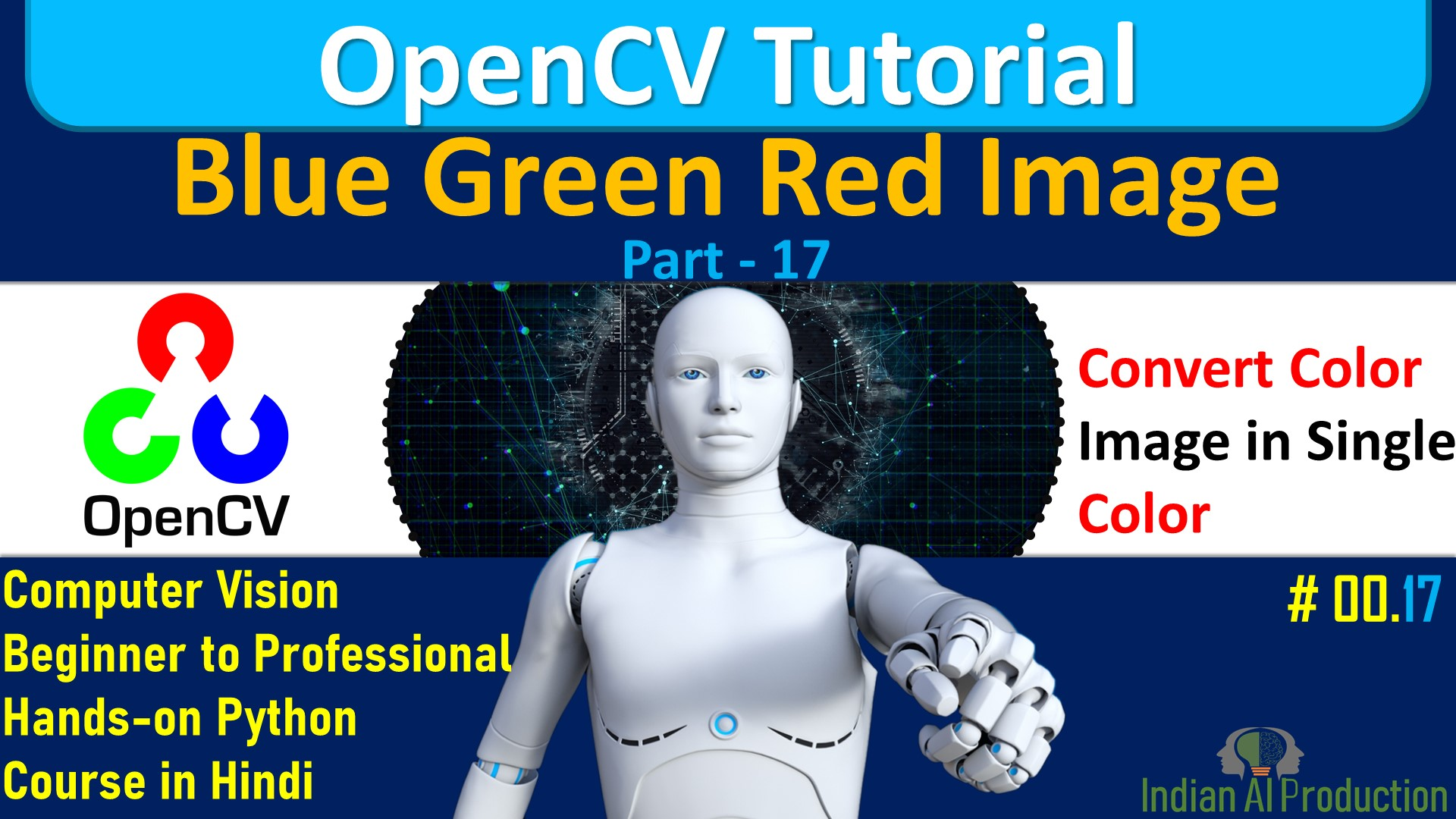## Conver Color or Gray Image into Blue Green Red image using OpenCV Python

In Python OpenCV Tutorial, Explained How to Conver Color or Gray Image into Blue Green Red image using OpenCV Python. Get the answers of below questions: How do I change the color of an image in OpenCV?How to extract red, green, blue color from an image in pythonGreen channel extraction in image processing pythonWhat are channels in OpenCV?How do I merge an image in OpenCV? Code(Jupyter...## Split & Merge Image Using OpenCV Python

In Python OpenCV Tutorial, Explained How to split and merge image using numpy indexing and python OpenCV cv2.split()  & cv2.merge() function? Syntax: cv2.split(m ) -> mv Parameters: . @overload . @param m input multi-channel array. . @param mv output vector of arrays; the arrays themselves are reallocated, if needed. Syntax: cv2.merge(mv ) -> dst Parameters: . @param mv input vector of...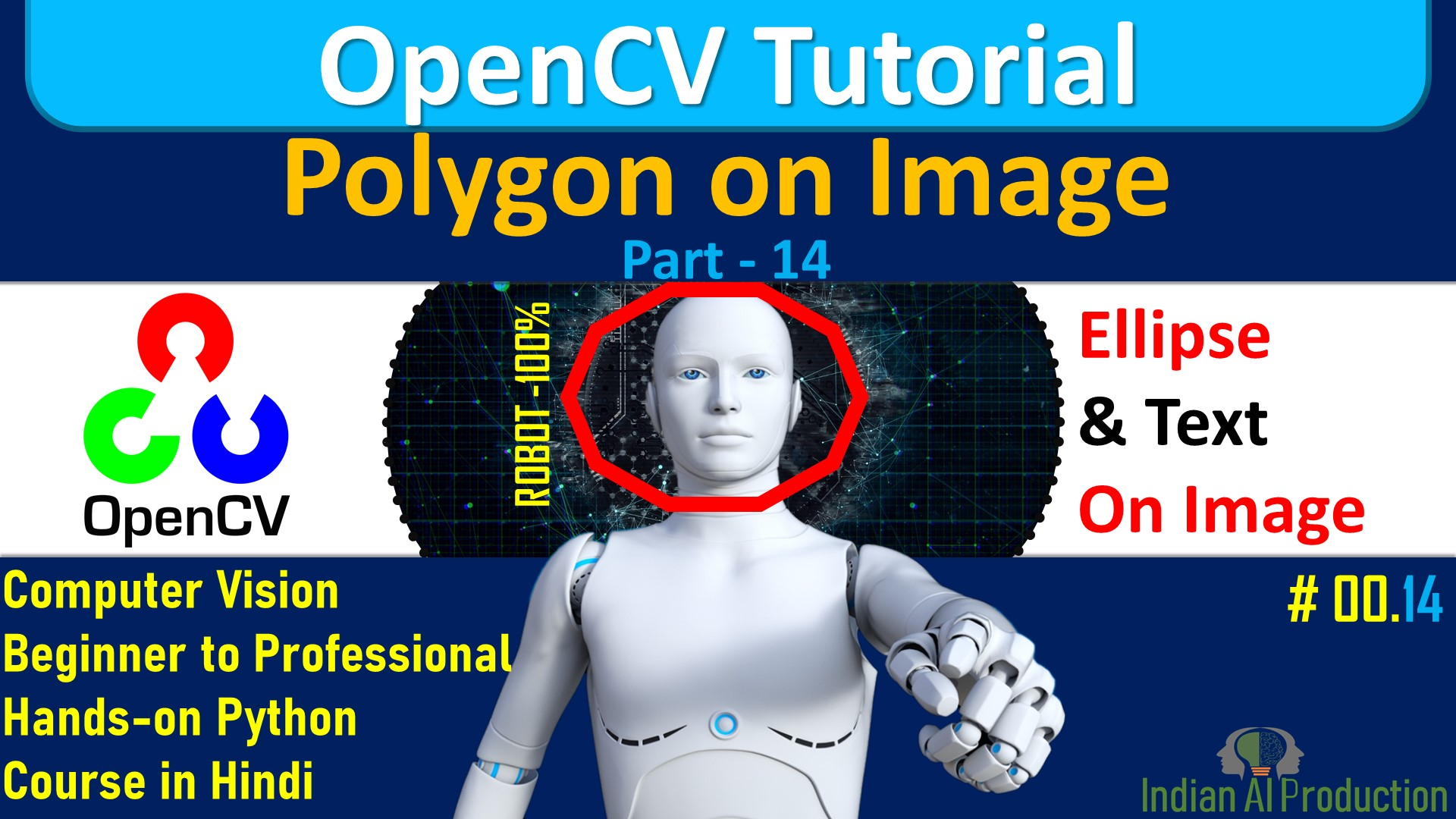## Draw Polygons On An Image using OpenCV Python

In Python OpenCV Tutorial, Explained How to put text and Polylines over the image using python OpenCV cv2.polylines() function? Syntax: cv2.polylines(img, pts, isClosed, color ]])Return: Image with Polygon Parameters: . @param img Image. . @param pts Array of polygonal curves. . @param isClosed Flag indicating whether the drawn polylines are closed or not. If they are closed, ....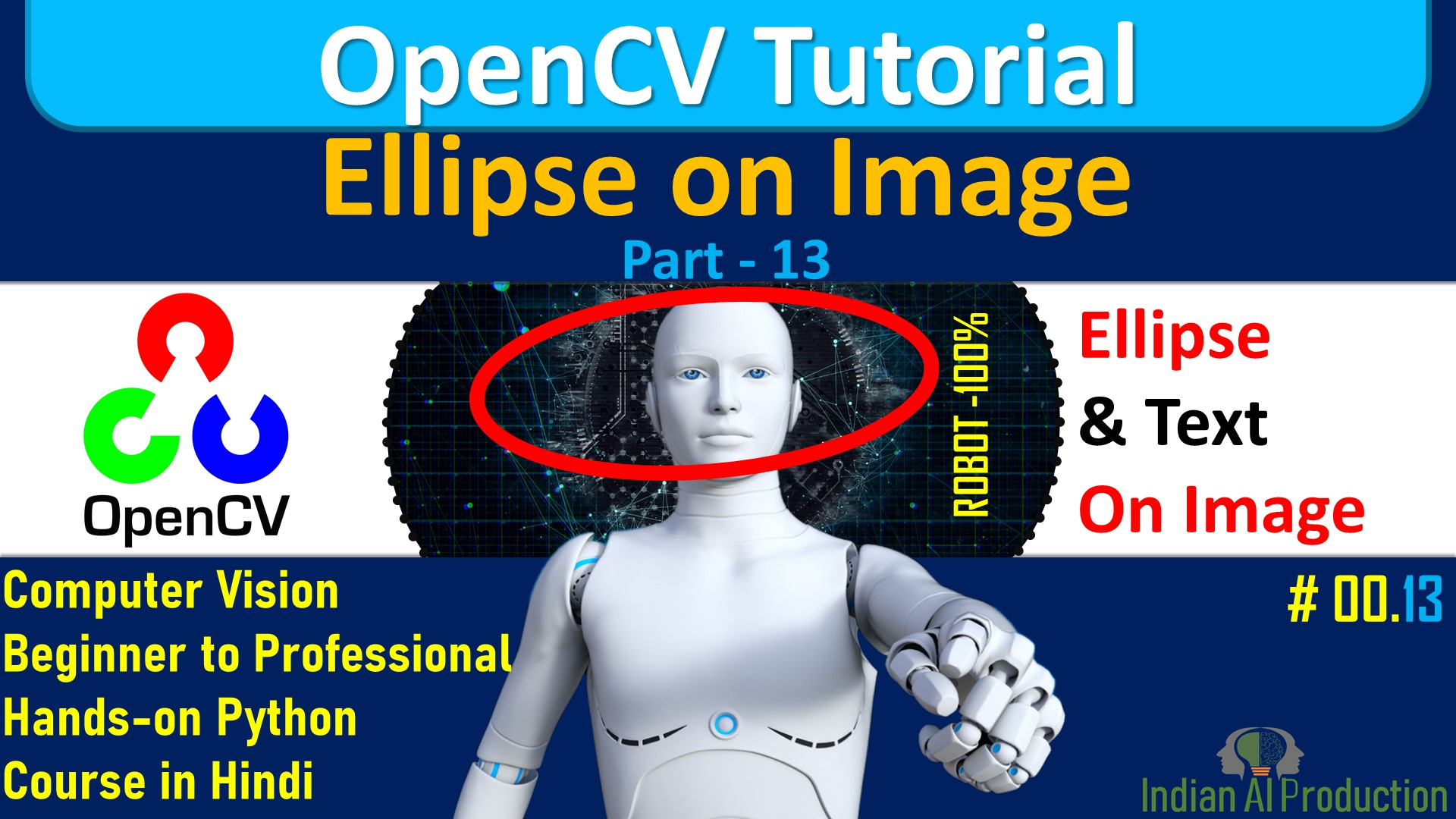## Draw Ellipse on NumPy array and Image Using OpenCV Python

In Python OpenCV Tutorial, Explained How to put text and Ellipse over the image using python OpenCV cv2.ellipse() function? Syntax: cv2. ellipse(img, center, axes, angle, startAngle, endAngle, color ]])Return : Image with ellipse Parameters: . @param img Image. . @param center Center of the ellipse. . @param axes Half of the size of the ellipse main axes. . @param angle Ellipse rotation...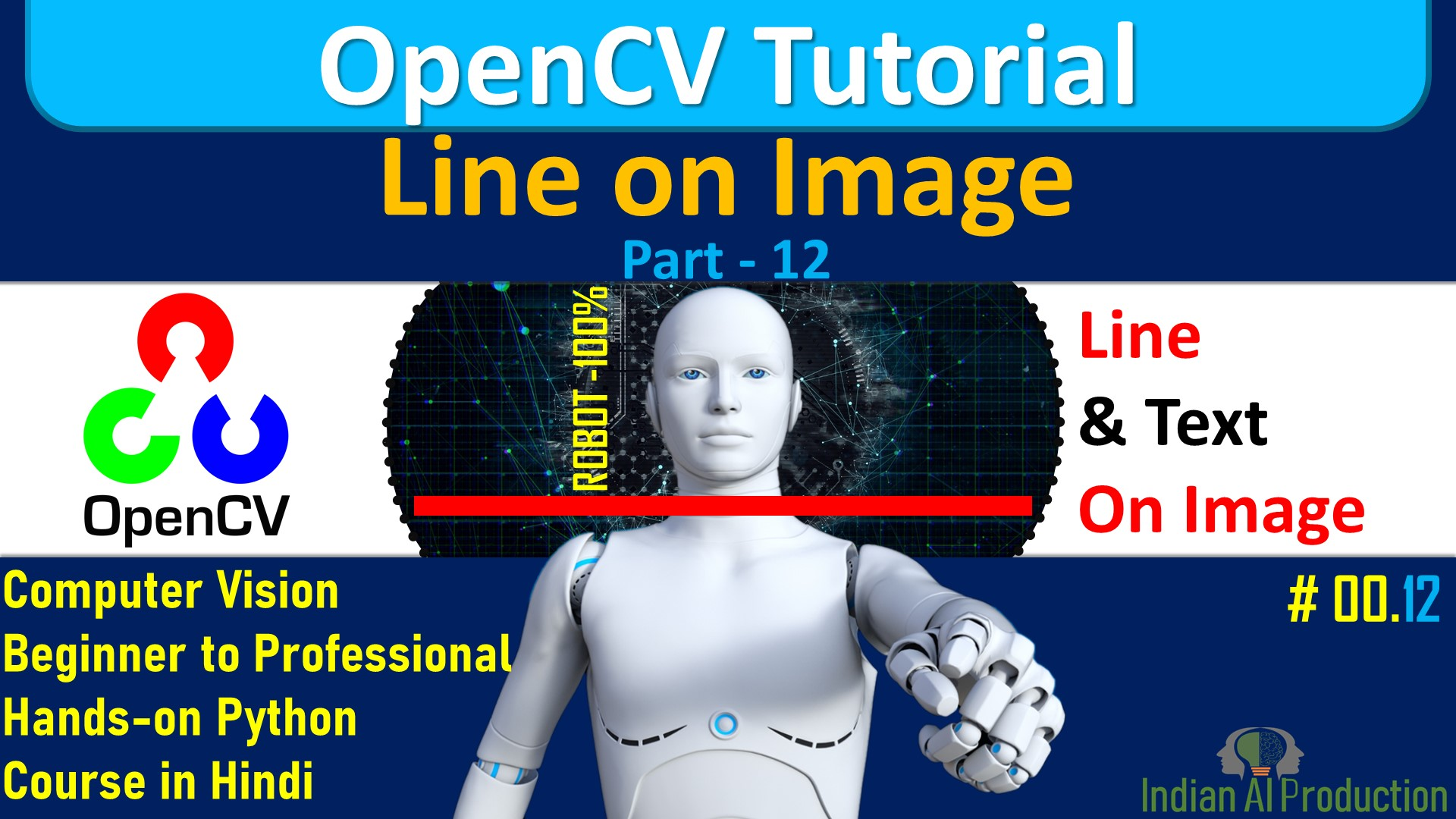## Draw Line, Print Text On An Image using OpenCV Python

In Python OpenCV Tutorial, Explained How to put text and Line over the image using python OpenCV cv2.line() function? Syntax: cv2.line(img, pt1, pt2, color ]]) Parameters: . @param img Image. . @param pt1 First point of the line segment. . @param pt2 Second point of the line segment. . @param color Line color. . @param thickness Line thickness. . @param lineType Type of the line. See...## Draw Circle, Print Text On An Image | OpenCV Tutorial

In Python OpenCV Tutorial, Explained How to put text and Circle over the image using python OpenCV? Syntax: cv2.circle(img, center, radius, color ]]) . The function cv::circle draws a simple or filled circle with a given center and radius. . @param img Image where the circle is drawn. . @param center Center of the circle. . @param radius Radius of the circle. . @param color Circle color. ....
Top